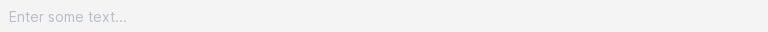# Textbox¶

Use textboxes to capture text inputs and passwords.

## Basic¶

Call `box()` with `mode='text'` to show a textbox.

The return value is the text entered into the box.

``````x = view(box(mode='text'))
view(f'You entered {x}.')
``````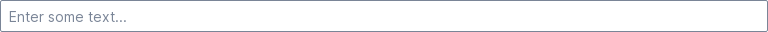## Set initial value¶

Set `value=` to prefill the box with a value.

`mode='text'` can be elided if `value=` is set.

``````speed = view(box(value='60 km/h'))
``````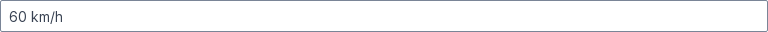## Set a label¶

Any text passed to `box()` is used as a label.

``````speed = view(box('Speed', value='60'))
``````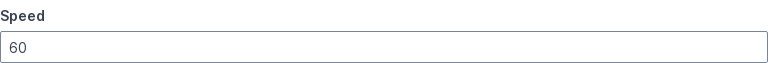## Show placeholder text¶

Use `placeholder=` to show placeholder text inside the box.

``````speed = view(box('Speed', placeholder='0 km/h'))
``````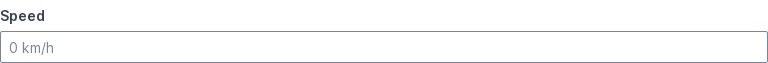## Mark as required¶

Add `required` to `mode` to indicate that input is required.

``````speed = view(box('Speed (km/h)', mode='required'))
``````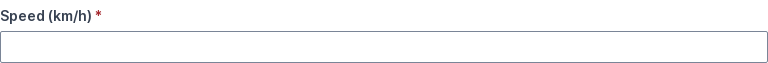## Control input format¶

Set `mask=` to specify an input mask. An input mask is used to format the text field for the expected entry.

For example, to accept a phone number, use an input mask containing three sets of digits.

``````phone = view(box('Phone', mask='(999) 999 - 9999'))
``````

• Use `a` to indicate a letter.
• Use `9` to indicate a number.
• Use `*` to indicate a letter or number.
• Use a backslash to escape any character.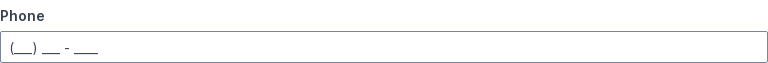## Show an icon¶

Set `icon=` to show an icon at the end of the box.

``````phrase = view(box('Filter results containing:', icon='Filter'))
view(f'You set a filter on `{phrase}`.')
``````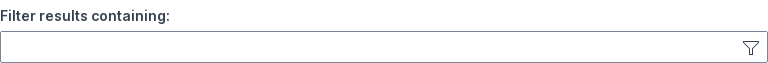## Set prefix text¶

Set `prefix=` to show a prefix at the start of the box.

``````website = view(box('Website', prefix='https://', value='example.com'))
``````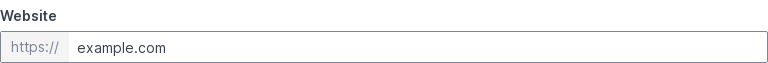## Set suffix text¶

Set `suffix=` to show a suffix at the end of the box.

``````website = view(box('Website', suffix='.com', value='example'))
``````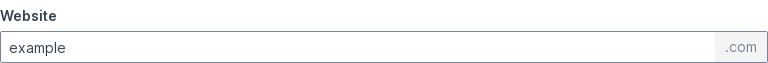## Set both prefix and suffix texts¶

A textbox can show both a prefix and a suffix at the same time.

``````website = view(box('Website', prefix='https://', suffix='.com', value='example'))
``````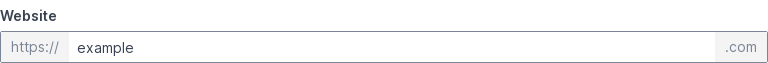## Show an error message¶

Set `error=` to show an error message below the box.

``````speed = view(box('Speed (km/h)', error='Invalid input'))
``````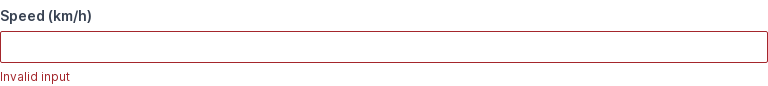Add `password` to `mode` when accepting passwords and other confidential inputs.

``````password = view(box('Password field', mode='password'))
``````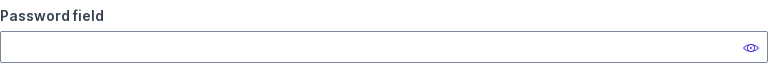## Enable multiple lines¶

Set `lines=` to show a multi-line text box (also called a text area).

``````bio = view(box('Bio:', lines=5))
view(f'**Bio:** {bio}')
``````

Note that multi-line textboxes can be resized by the user, and `lines=` only sets the initial height of the textbox.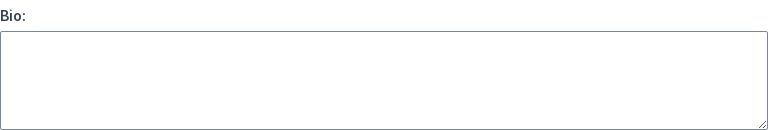## Disable¶

Set `disabled=True` to disable.

``````view(box(mode='text', disabled=True))
``````